Unit 1: Number Sense

# Topic E: More Counting

Practice your counting by filling in the counting chart. Have your instructor check your chart when you are done.

 0 1 2 3 4 5 6 7 8 9 10

If you had a pile of pennies or loonies, you would count by ones in order to find out how much money you have.

Use your counting chart and start at 1. Write down every second number.

 0 1 3 5

The numbers above are called odd numbers.

Use your counting chart and starting at 0. Write down every second number.

 0 2 4 6

The numbers above are called the even numbers. If you had a pile of toonies, you could count by twos to find out how much money you have.

Use your counting chart and start at 0. Count five and write down that number.

 0 5 10

If you had a pile of nickels or five dollar bills and wanted to know how much money you have, you would count by 5s.

Use your counting chart and starting at 0. Count ten and write down that number.

 0 10 20

If you had a pile of dimes or ten dollar bills and wanted to know how much money you have, you would count by 10s.

Exercise 1

Count how much money you have. Check your work using the answer key at the end of the exercise.

Example: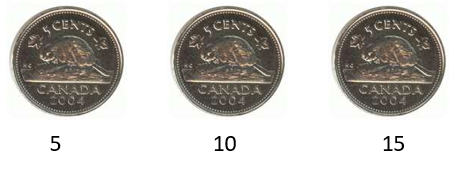How many nickels?      3

How much money do you have?    15 cents

1. How many toonies do you have?
How much money do you have?           dollars.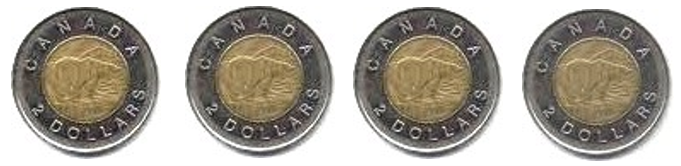2. How many dimes do you have?
How much money do you have?           cents.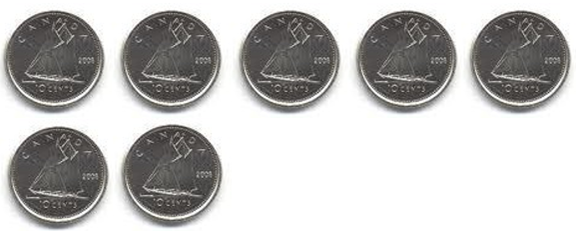3. How many nickels do you have?
How much money do you have?           cents.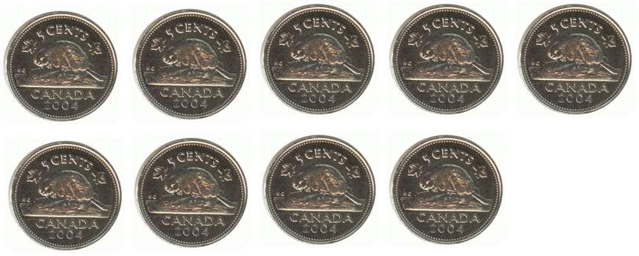4. How many dimes do you have?
How much money do you have?           cents.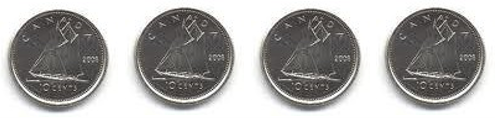5. How many nickels do you have?
How much money do you have?           cents.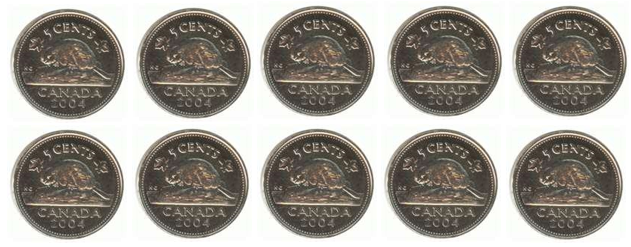6. How many toonies do you have?
How much money do you have?           dollars.7. How many dimes do you have?
How much money do you have?           cents.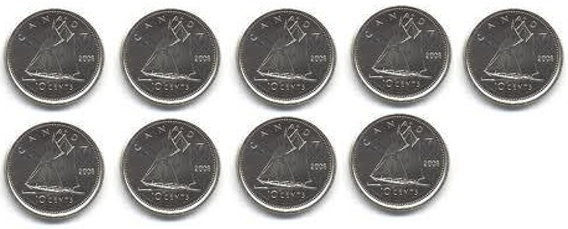1. 4 toonies, 8 dollars
2. 7 dimes, 70 cents
3. 9 nickels, 45 cents
4. 4 dimes, 40 cents
5. 10 nickels, 50 cents
6. 13 toonies, 26 dollars
7. 9 dimes, 90 cents

# Topic E Self-Test

Mark   /16             Aim    13/16

1. Write the first 10 odd numbers starting with 1. (5 marks)
2. Write the first 10 even numbers starting at 2. (5 marks)
3. How much money do you have? (6 marks, 2 marks each)
1. How much money do you have?           cents.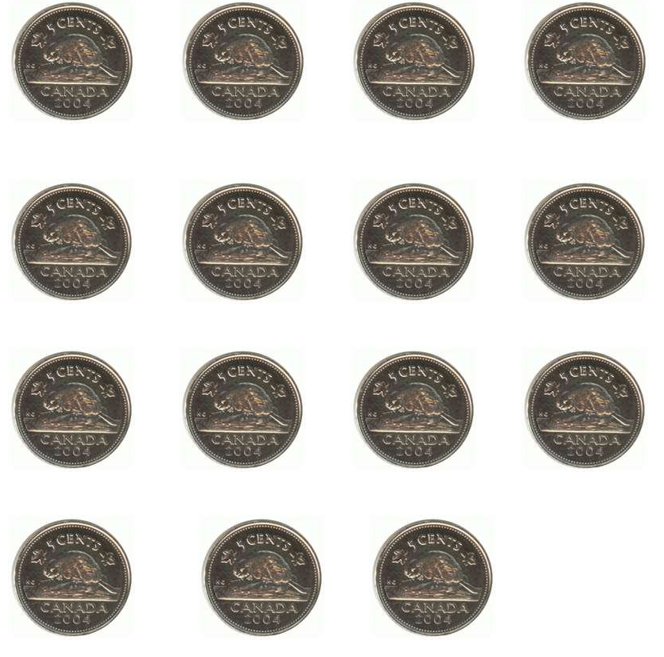2. How much money do you have?           dollars.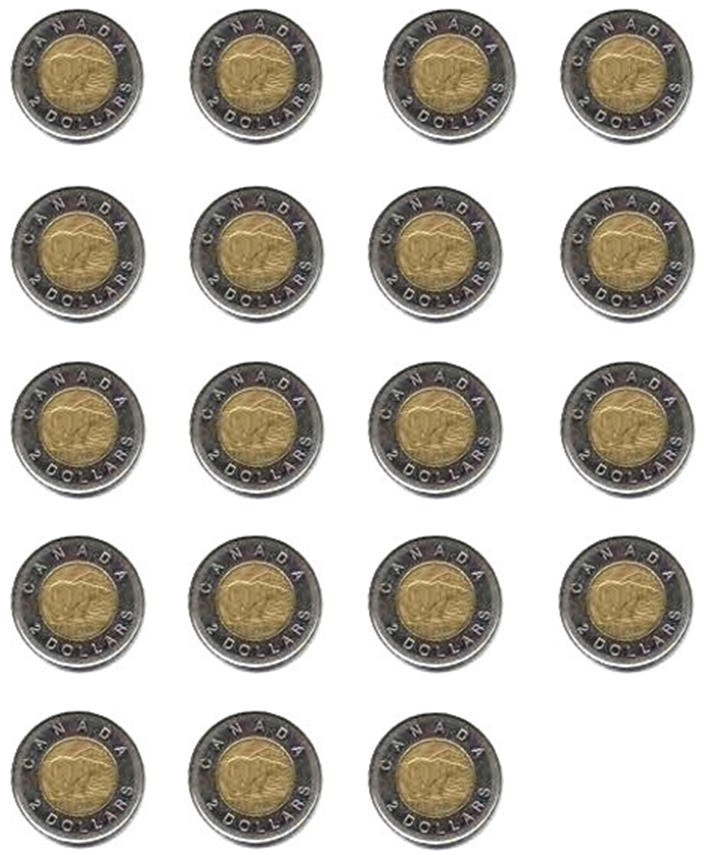3. How much money do you have?           cents.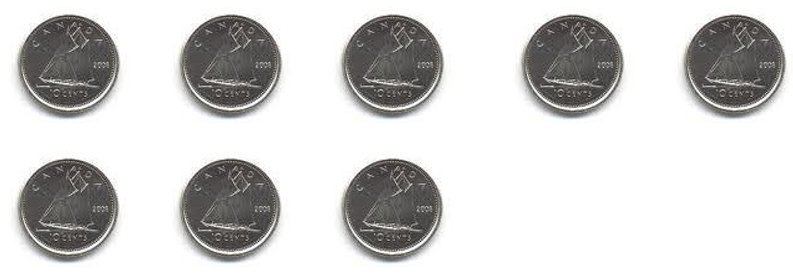1. 1, 3, 5, 7, 9, 11, 13, 15 17, 19
1. 2, 4, 6, 8, 10, 12, 14, 16, 18, 20
1. 75 cents
2. 38 dollars
3. 80 cents

Emotions Check

How are you feeling?